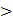# The magnetic field associated with a light wave is given, at the origin, by B=B0[sin(3.14107)ct + sin(6.287ct).  If this light gals on a silver plate having a work function of 4.7 eV, What will be the maximum kinetic energy of the photo electrons? (c=3108ms−1,h=6.610−34 J-s) a. 7.72eV b. 8.52eV c. 12.5eV d. 6.82eV

## Question ID - 50156 :- The magnetic field associated with a light wave is given, at the origin, by B=B0[sin(3.14107)ct + sin(6.287ct).  If this light gals on a silver plate having a work function of 4.7 eV, What will be the maximum kinetic energy of the photo electrons? (c=3108ms−1,h=6.610−34 J-s) a. 7.72eV b. 8.52eV c. 12.5eV d. 6.82eV

3537

B1=B0sin(107C)t + B0 sin(210−7C)t since there are two EM waves with different frequency, to get maximum kinetic energy we take the photon with higher frequency

B1=B0sin(107C)t  V1=B2=B0sin(2107C)t  V2= 10C

Where C is speed of light C=310m/s

V2V1

So KE of photoelectron will be maximum for photon of higher energy.

V2 =107CHz

hv=+KEmax

energy of photon

Eph=hv=6.610−3410−73109

Eph=6.6310−19J

=KEmax =Eph=12.375−4.7

=7.675eV7.7 eV

Next Question :
 Let ABCD be a parallelogram and ABEF be a rectangle with EF lying along the line C. If AB = 7 cm and BE = 6.5 cm, then the area of parallelogram is (a) 11.375 cm2 (b) 22.75 cm2 (c) 45 cm2 (d) 45.5 cm2